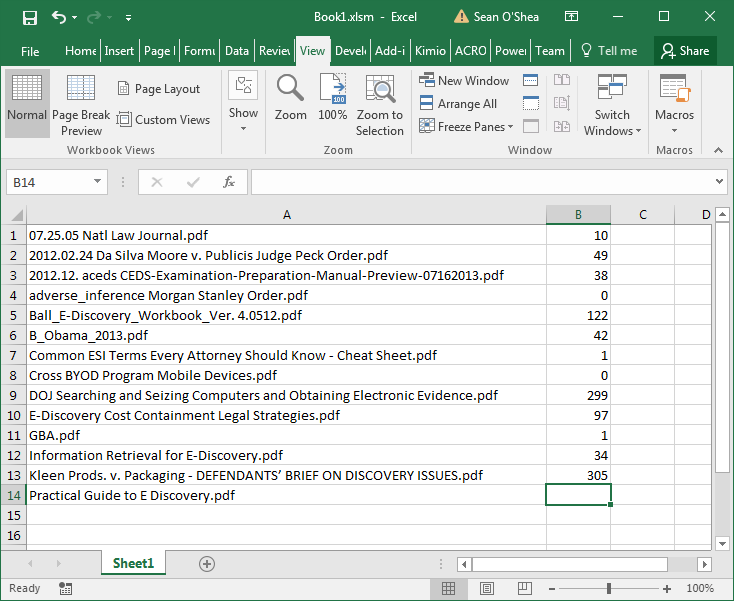Search

# UPDATE - Macro to Get Page Count of PDF Files

Here's an update to my tip of a couple of nights ago, where I posted VBA code for Excel that can be used to get the page counts of PDFs in a specified folder. I have been using that code at work and noticed that it doesn't always give the correct page counts.

In the same forum, Ralajer posted a different VBA code to accomplish the same task. I tested it on a random sample of PDFs from my PC, and while it didn't work perfectly, it did have an advantage over the code posted on April 11. The prior code will unaccountably give incorrect page counts that can be off by many pages. This code will either give a count of 0 if it can't determine the page count, or the VBA code will give you a run time error if the folder has a PDF it can't process at all. At least with this code you are alerted when there's a problem. For the PDFs that it does give a non-zero count for, I did not find any mistakes.Don't forget the Tip of the Night for June 29, 2015 when I posted about a free utility that will give you a list showing PDF page counts.Sub PDFandNumPages() Dim Folder As Object Dim file As Object Dim fso As Object Dim iExtLen As Integer, iRow As Integer Dim sFolder As String, sExt As String Dim sPDFName As String

sExt = "pdf" iExtLen = Len(sExt) iRow = 1 ' Must have a '\' at the end of path sFolder = "C:\pdf_Directory\" Set fso = CreateObject("Scripting.FileSystemObject") If sFolder <> "" Then Set Folder = fso.GetFolder(sFolder) For Each file In Folder.Files If Right(file, iExtLen) = sExt Then Cells(iRow, 1).Value = file.Name Cells(iRow, 2).Value = pageCount(sFolder & file.Name) iRow = iRow + 1 End If Next file End If

End Sub

Function pageCount(sFilePathName As String) As Integer

Dim nFileNum As Integer Dim sInput As String Dim sNumPages As String Dim iPosN1 As Integer, iPosN2 As Integer Dim iPosCount1 As Integer, iPosCount2 As Integer Dim iEndsearch As Integer

' Get an available file number from the system nFileNum = FreeFile

'OPEN the PDF file in Binary mode Open sFilePathName For Binary Lock Read Write As #nFileNum ' Get the data from the file Do Until EOF(nFileNum) Input #1, sInput sInput = UCase(sInput) iPosN1 = InStr(1, sInput, "/N ") + 3 iPosN2 = InStr(iPosN1, sInput, "/") iPosCount1 = InStr(1, sInput, "/COUNT ") + 7 iPosCount2 = InStr(iPosCount1, sInput, "/") If iPosN1 > 3 Then sNumPages = Mid(sInput, iPosN1, iPosN2 - iPosN1) Exit Do ElseIf iPosCount1 > 7 Then sNumPages = Mid(sInput, iPosCount1, iPosCount2 - iPosCount1) Exit Do ' Prevent overflow and assigns 0 to number of pages if strings are not in binary ElseIf iEndsearch > 1001 Then sNumPages = "0" Exit Do End If iEndsearch = iEndsearch + 1 Loop ' Close pdf file Close #nFileNum pageCount = CInt(sNumPages) End Function